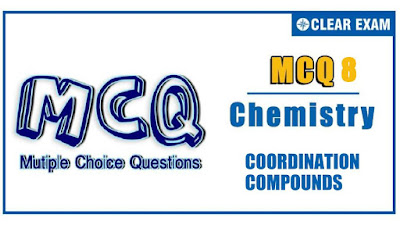## [LATEST]\$type=sticky\$show=home\$rm=0\$va=0\$count=4\$va=0

JEE Advanced is a high level exam that checks your concept by putting various types of questions. The questions are in a different format from JEE mains, herein the questions are : single correct multiple choice questions, multiple correct multiple choice questions, statement based questions and comprehension type questions..

Q1.
In the complex [Pt(O_2 ) (en)_2 (Br)]^(2+) coordination number and oxidation number of platinum are
•  4,3
•  4,5
•  4,6
•  6,4
Solution
(c)Superoxide (-1)

[Pt(O_2 ) (en)_2 (Br)]^(2+)

Bidentate
Thus, ON=+4
CN=6

Q2.
Which of the following types of bonds are present in CuSO_4∙5H_2 O
I. Electrovalent II. Covalent III. Coordinate
Select the correct answer out of
•  I,II
•  II,III
•  I,III
•  I,II,III
Solution
(d) CuSO_4∙5H_2 Oexists as[Cu(H_2 O)_4 ]SO_4∙H_2 O Ionic bonding between [Cu(H_2 O)_4 ]^(2+) and SO_4^(2-) Covalent bonding in SO_4^(2-) and H_2 Ocoordinate bonding in complex between Cu^(2+) and H_2 O

Q3. EAN of the elements (*) are equal in
•  Ni(CO)_4,[Fe(CN)_6 ]^(4-)
•  [Ni(en)_2 ]^(2+),[Fe(H_2 O)_6 ]^(2+)
•  [Co(CN)_6 ]^(3-),[Fe(CN)_6 ]^(3-)
•  [Ni(en)_2 ]^(2+),[Sc(H_2 O)_6 ]^(3+)
Solution
(a)

Q4. Match the geometry (given in Column I) with the complexes (in Column II) in
Column I Column II
A. Octahedral [Ni(CN)_4 ]^(2-)
B. Square planar Ni(CO)_4
C. Tetrahedral [Fe(CN)_6 ]^(4-)
A B C
•  1 2 3
•  3 1 2
•  3 2 1
•  2 1 3
Solution
(b)
(A) octanehdral (3) [Fe(CN)_6 ]^(4-) d^2 sp^3
(B) square planar (1) [Ni(CN)_4 ]^(2-) dsp^2
(C) Tetrahedral (2) Ni(CO)_4 sp^3

Q5.The compound(s) that exhibit (s) geometrical isomerism is (are)
•  [Pt(en)Cl_2]
•  [Pt(en)_2 Cl_2
•  [Pt(en)_2 Cl_2 ]Cl_2
•  [Pt(NH_3 )_4 Cl_2
Solution
(c)

Q6. What is the ratio of uncomplexed to complexed Zn^(2+) ion in a solution that is 10 M in , in NH_3if the stability constant of is [Zn(NH_3 )_4 ]^(2+ )is 3 ×10^9
•  3.3 ×10^(-9)
•  3.3 ×10^(-11)
• 3.3 ×10^(-14)
•  3 ×10^(-13)
Solution
(c)

Q7.Complexes of which ions are not kinetically labile
•  Cu^(2+),Ni^(2+)
•  Cr^(3+),Co^(3+)
•  V^(3+),Ti^(4+)
•  None of the above is correct
Solution
(b)

Q8.In which case racemic mixture is obtained on mixing its mirror images in 1:1 molar ratio
•  [Cr(en)_3 ]^(3+)
•  [Ni(DMG)_2]
•  cis-[Cu(Gly)_2]
•  In all cases
Solution
(a) [Cr(en)_3 ]^(3+) exists as enantiomer and thus 1 : 1mixture of two optically active forms results in the formation of recemic mixture

Q9.Which of the following an example of coordination compound
•  CoCl_3∙6NH_3
•  CoCl_3∙6NH_3
•  FeSO_4∙6H_2 O
•  FeSO_4∙(NH_4 )_2 SO_4∙6H_2 O
Solution
(a)
1. [CO(NH_3 )_6 ]Cl_3
2. Double salt
3. Simple salt
4. Double salt

Q10. Which is the incorrect observation
•  For the complex , [CoF_6 ]^(3-) ,F is a weak-field ligand, so that ∆_oct is less than P (electron –pairing energy) and is thus high spin complex
•  For the complex , [Co(NH_3 )_6 ]^(3+),NH_3is a strong-field ligand, so that ∆_oct is greater than P and thus low spin complex
•  ∆_t= +4/9 ∆_oct
• Greater the ionic charge on the central metal ion the greater the value of ∆
Solution
(c)#### Written by: AUTHORNAME

AUTHORDESCRIPTION## Want to know more

Please fill in the details below:

## Latest NEET Articles\$type=three\$c=3\$author=hide\$comment=hide\$rm=hide\$date=hide\$snippet=hide

Name

ltr
item
BEST NEET COACHING CENTER | BEST IIT JEE COACHING INSTITUTE | BEST NEET & IIT JEE COACHING: Coordination Compounds - Quiz 8
Coordination Compounds - Quiz 8
https://1.bp.blogspot.com/-yODRX607Nck/YOiQ72aX1XI/AAAAAAAAVis/qkzoHcB4SDUnJ4Ly3c1uT-Ql8tY0buRaACLcBGAsYHQ/s400/8.jpg
https://1.bp.blogspot.com/-yODRX607Nck/YOiQ72aX1XI/AAAAAAAAVis/qkzoHcB4SDUnJ4Ly3c1uT-Ql8tY0buRaACLcBGAsYHQ/s72-c/8.jpg
BEST NEET COACHING CENTER | BEST IIT JEE COACHING INSTITUTE | BEST NEET & IIT JEE COACHING
https://www.cleariitmedical.com/2021/07/coordination-compounds-quiz-8.html
https://www.cleariitmedical.com/
https://www.cleariitmedical.com/
https://www.cleariitmedical.com/2021/07/coordination-compounds-quiz-8.html
true
7783647550433378923
UTF-8

STAY CONNECTED